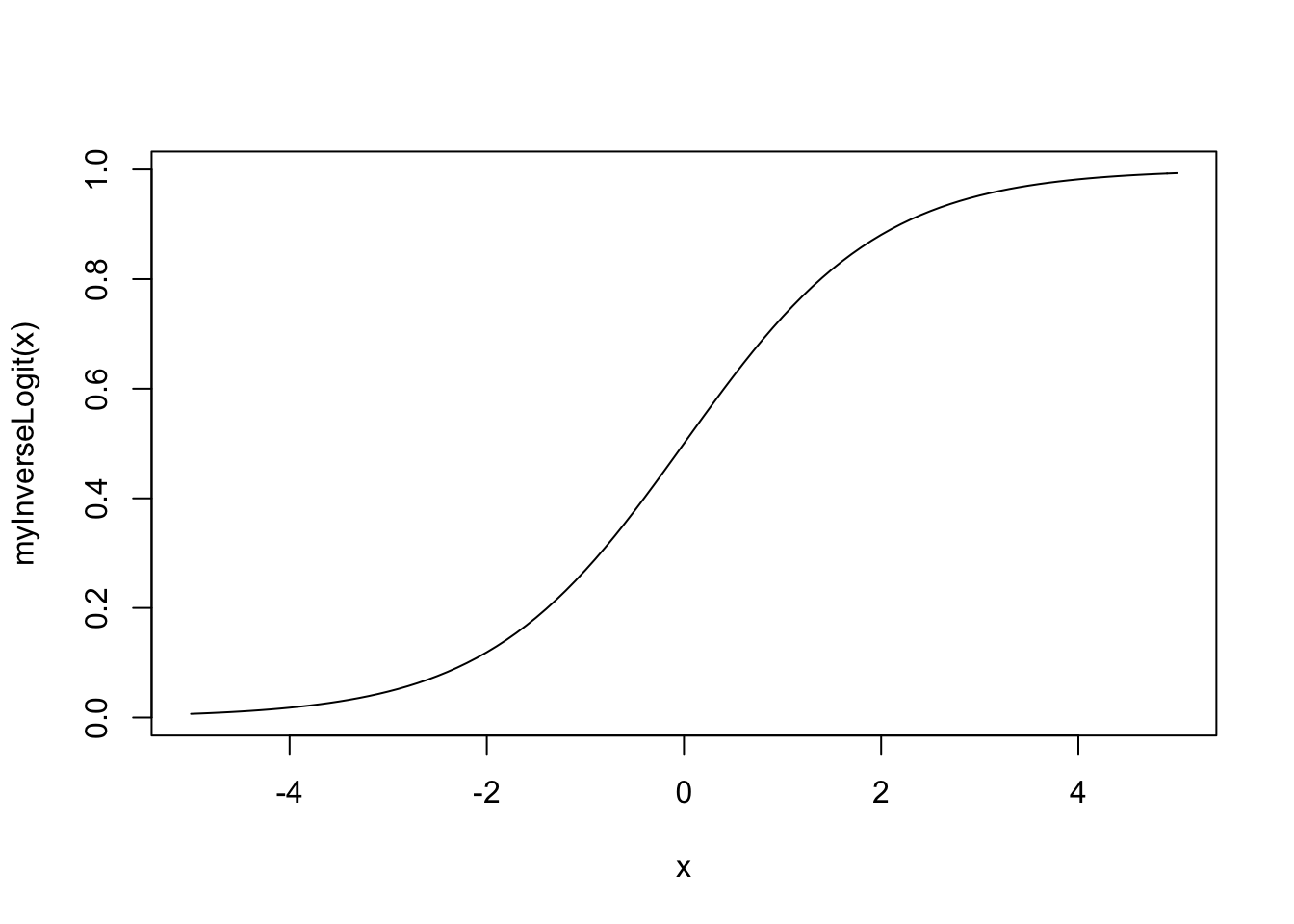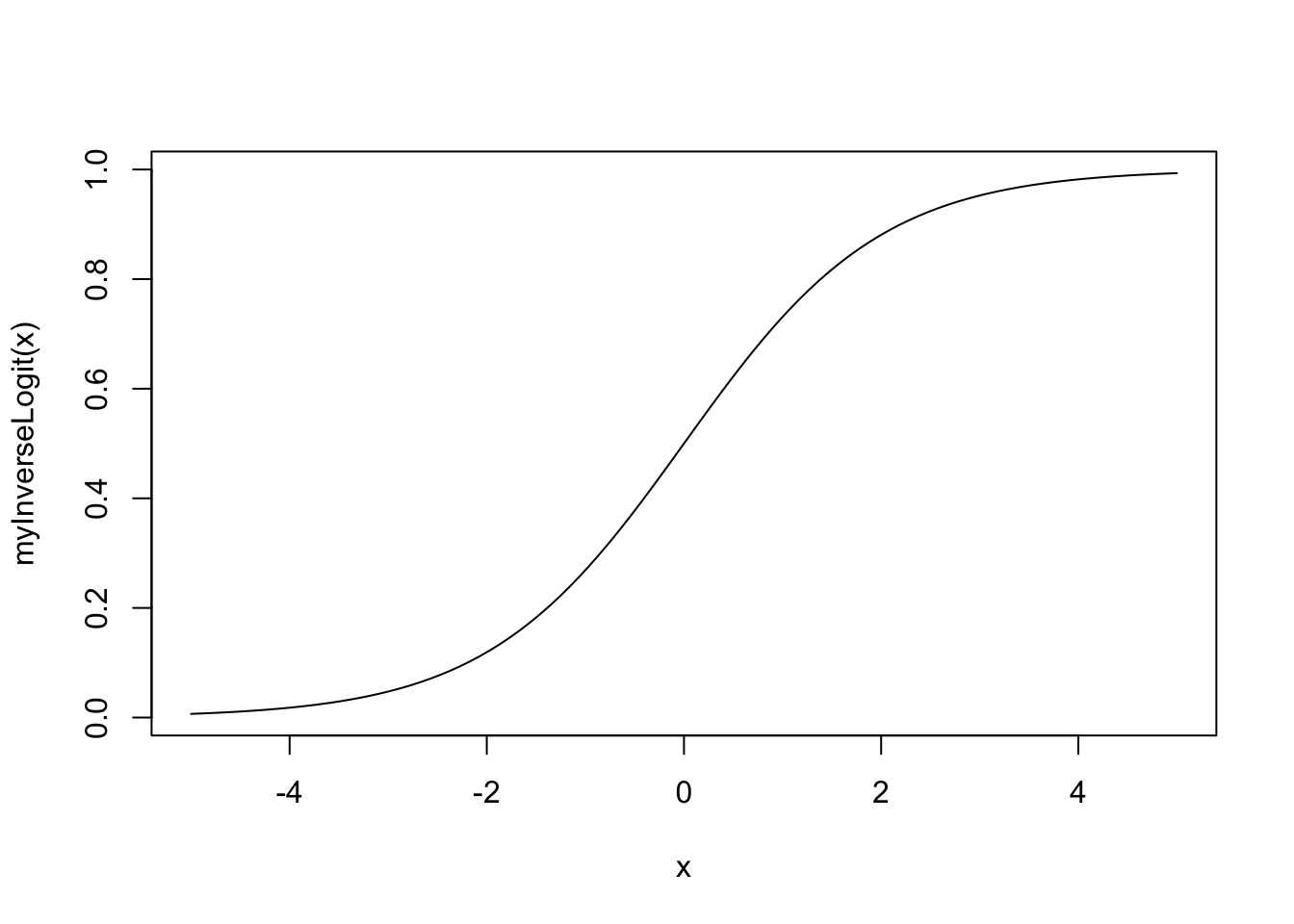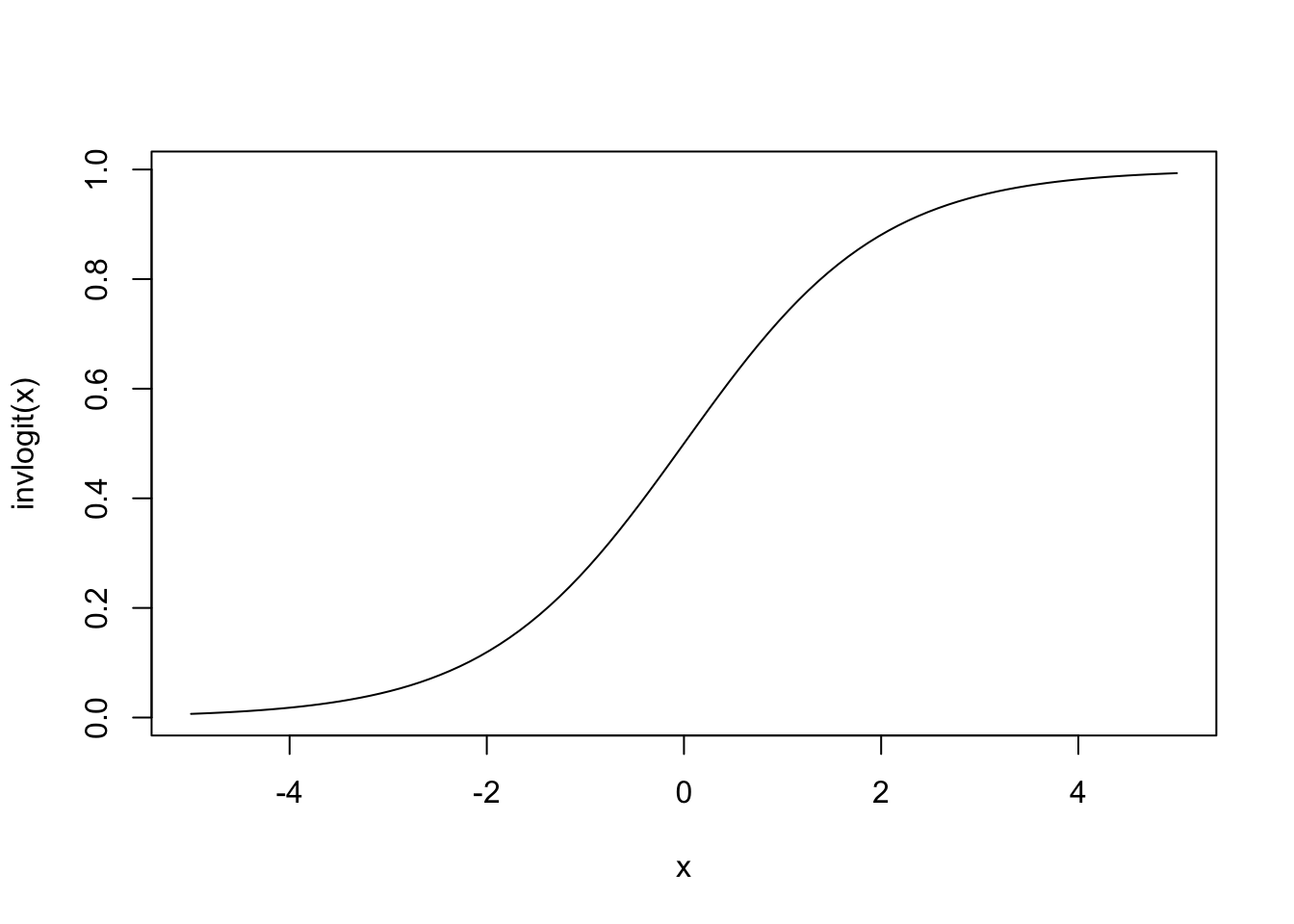# Introduction

Hello everyone! Here is a chance to explore some of the code and concepts covered in your QS903 lectures.

Specifically, we cover:

• The curve function
• The %*% operator
• The proportional odds model (for ordered categorical outcomes)
• The multinomial model (for unordered categorical outcomes)

You have also covered the multi-level models. These are cool and exciting! However, we are limited to two hours. So this workshop focuses on the models you may use in your first assignments.

# The curve function

Imagine you want to plot a function. One way to do this is to define a function,

``````myInverseLogit <- function(x){
exp(x)/(1+exp(x)) # this is the inverse logit function, btw
}``````

generate some value from the function

``````x <- seq(from = -5, to = 5, by = 0.1)
y <- myInverseLogit(x)``````

and then plot a line using those values

``plot(x, y, type = 'l', ylab = 'myInverseLogit(x)') # type is line 'l'``The curve function combines generating predictions and plotting a line into one command.

``curve(expr = myInverseLogit, from = -5, to = 5, n = 100)``Nice. You may remember using the inverse logit function for the logit model. The invlogit function from the arm package you gives us the same predictions.

``require(arm)``
``## Loading required package: arm``
``## Loading required package: MASS``
``## Loading required package: Matrix``
``## Loading required package: lme4``
``````##
## arm (Version 1.10-1, built: 2018-4-12)``````
``## Working directory is /Users/jamestripp/Desktop``
``curve(expr = invlogit, from = -5, to = 5, n = 100)``An interesting feature of curve is that it is easy to overlay a curve onto a base R plot.

``````x <- seq(from = -5, to = 5, by = 1)
plot(x = x, y = invlogit(x))
curve(expr = invlogit, from = -5, to = 5, n = 100, add = TRUE, col = 'pink') # note add is TRUE``````